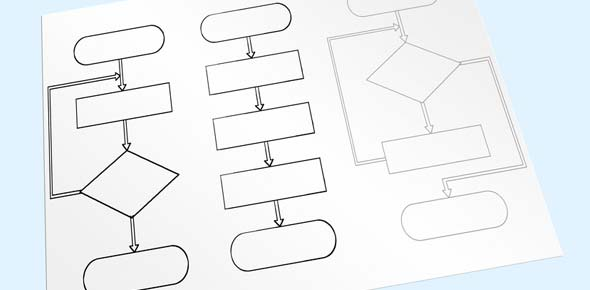# Intro To Logs Quiz 1

14 Questions | Total Attempts: 83Settings• 1.
Write the equation 53 = 125 in log form.
• A.

Log 3 (125) = 5

• B.

Log 125 (5) = 3

• C.

Log 5 (125) = 3

• D.

Log 5 (3) = 124

• 2.
Write the log equation log 2 32 = 5 in exponential form
• A.

2 ^ 5 = 32

• B.

5 ^ 2 = 32

• C.

2 ^ 32 = 5

• D.

32 ^ 2 = 5

• 3.
Write the equation a b = x in log form
• A.

Log b (x) = a

• B.

Log a (x) = b

• C.

Log b (a) = x

• D.

Log x (a) = b

• 4.
The equation 2 x = 9 can be entered in the calculator in which form?
• A.

(log 9) / (log 2)

• B.

Log 9 * log 2

• C.

(log 9 )^2

• D.

(log 2) / (log 9)

• 5.
A logarithmic function is the inverse of which function?
• A.

• B.

Linear

• C.

Cubic

• D.

Exponential

• 6.
Solve . Round to 4 decimal places.
• A.

0.7442

• B.

1.3437

• C.

0.4472

• D.

-0.4472

• 7.
Solve
• A.

15

• B.

125

• C.

1.465

• D.

0.6826

• 8.
Condense using log properties then simplify
• A.

2

• B.

3

• C.

4

• D.

8

• 9.
Solve for x.
• A.

3

• B.

9

• C.

27

• D.

1

• 10.
Solve the following equation and select one of the answer choices.
• A.

30

• B.

3

• C.

10

• D.

Log10

• 11.
5−x =  1/25Solve for x.
• A.

-2

• B.

1/2

• C.

3

• D.

-1

• E.

2

• 12.
Log ab cExpand this logarithmic expression.
• A.

Log a + log b − log c

• B.

Ab/c

• C.

Log a x log b / log c

• D.

Log a + log b + log c

• E.

Log a - log b + log c

• 13.
Log a  +   log b  +   log cCondense this logarithmic expression.
• A.

Log a+b+c

• B.

Log abc

• C.

Log a + b + c

• 14.
Write the equation in logarithmic form: 44=256
• A.

Log256 4=4

• B.

4log 256=4

• C.

256log 4=4

• D.

Log4 256=4

Related TopicsBack to top